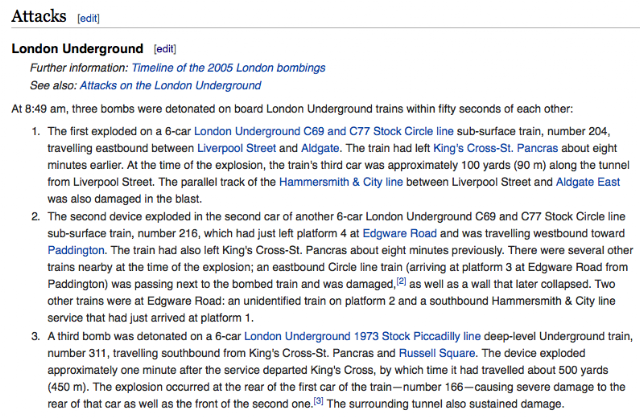## Tuesday, May 26, 2015

### 777 | London Train Bombings & Philadelphia Train Crash, #1888:49, or 11 'til 9?
July 7 is the 188th day of the year, much like Train #188 crashing in Philadelphia.  The bombing was at 8:49, or 11 'til 9, on an extra special date in numerology.
• 7/7/2005 >>>> 7/7/(2+0+0+5) = 7/7/7
• C69 >>>>> 369
• C77 >>>>>377
Below are the Gematria of the names from the incident.
• Liverpool = 3+9+4+5+9+7+6+6+3 = 52
• Street = 1+2+9+5+5+2 = 24/33
• Liverpool Street = 76/85
• Aldgate = 1+3+4+7+1+2+5 = 23
• East  = 5+1+1+2 = 9/18
• Aldgate East = 32/41
• King's 2+9+5+7+1 = 24/33
• Cross = 3+9+6+1+1 = 20/38
• King's Cross = 44/71
• St. Pancras = 1+2+7+1+5+3+9+1+1 = 30/39/48
• Hammersmith = 8+1+4+4+5+9+1+4+9+2+8 = 55/64
• City Line = 3+9+2+7+3+9+5+5 = 43
• Edgware = 5+4+7+5+1+9+5 = 36
• Road = 9+6+1+4 = 20
• Paddington = 7+1+4+4+9+5+7+2+6+5 = 50
• London = 3+6+5+4+6+5 = 29
• Underground = 3+5+4+5+9+7+9+6+3+5+4 = 60
• London Underground = 89
• Stock = 1+2+6+3+2 = 14/23
• Piccadilly = 7+1+3+3+1+4+9+3+3+7 = 49
• Line = 3+9+5+5 = 22
• Stock Piccadilly Line = 85/94
• Russell = 9+3+1+1+5+3+3 = 25/43
• Square = 1+8+3+1+9+5 = 27/36
• Russell Square = 52/79
Notice the London Underground Line was #311.  It was from the year 1973, emphasis on '73.  The bomb exploded at the rear of Car #166.  (Twin Towers = 166 in Simple English Gematria, so does 'September Eleven')

More noteworthy Gematria below.
• Number = 5+3+4+2+5+9 = 28
• 30
• Double = 4+6+3+2+3+5 = 23
• Decker = 4+5+3+2+5+9 = 28
• Bus = 2+3+1 = 7/16
• Double Decker = 61
• Double Decker Bus = 68/77
• Number Double Decker = 89
• Number Double Decker Bus = 96/105
• Number 30 Double Decker = 119
• Number 30 Double Decker Bus = 125/134
• Dennis = 4+5+5+5+9+1 = 29/38
• Trident = 2+9+9+4+5+5+2 = 36
• Dennis Trident = 65/74
• Stagecoach = 1+2+1+7+5+3+6+1+3+8 = 37/46
• London = 3+6+5+4+6+5 = 29
• Stagecoach London = 66/75
• Marble = 4+1+9+2+3+5 = 24
• Arch = 1+9+3+8 = 21
• Marble Arch = 45
• Hackney  = 8+1+3+2+5+5+7 = 31
• Wick = 5+9+3+2 = 19
• Hackney Wick = 50
• Tavistock  = 2+1+4+9+1+2+6+3+2 = 30/39
• Square = 1+8+3+1+9+5 = 27/36
• Tavistock Square 57/66/75
Notice the explosion was at 9:47.
• 9
• 47 = 4+7 = 11
• 9:47 or 9:11 in numerology
• Just like how the first bomb explosion in the bobming series is at 8:49, or 11 'til 9.
Also notice the BBC and 'The Sun' broke the news.
• BBC = 223
• The Sun = 2+8+5+1+3+5 = 24/33